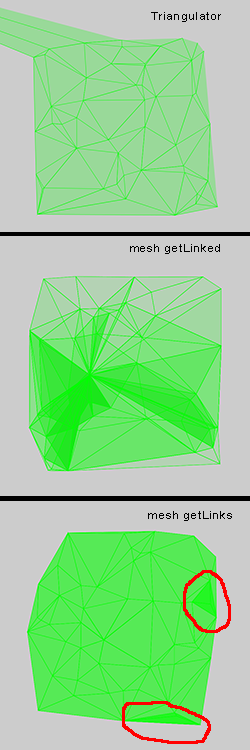#### Howdy, Stranger!

We are about to switch to a new forum software. Until then we have removed the registration on this forum.

# Delaunay Triangles

I want to get Delaunay triangles from an array of points.I tried using the code I found here: https://github.com/GlitchTools/Atagen/blob/master/GRDelaunay/Triangulator.pde

That gave me my triangles but also always added one extra point stretching off outside the canvas!

Lee Byron's mesh library seemed like it might be a better way to go http://leebyron.com/mesh/

However, the Delaunay class in that library doesn't actually return triangles or groups of three points - just links between pairs of indices in the points array.

I tried using getLinked() to find the points that link to each point, but that was a mess. My guess is that while the numbers it returns are indices to the original array, the number it takes as a parameter is not.

Finally I tried using getLinks(), and nested loops to search through all the links to find matches.

``````   ArrayList<Triangle> triangles  = new ArrayList<Triangle>();  //the Triangle class just stores 3 PVectors

for (int a = 0; a < myLinks.length; a++) { // loop through all links

for (int b = 0; b < myLinks.length; b++) { //loop through all links again...

//...except a
if (!(b == a)){

//Links that connect to the starting point could be either way round

//if start of b connects to start of a

for (int c = 0; c < myLinks.length; c++) {

//And the remaining link could be either way round

Triangle foundTri = new Triangle();
foundTri.p1 = new PVector(floatPoints[aStart], floatPoints[aStart]);
foundTri.p3 = new PVector(floatPoints[aEnd], floatPoints[aEnd]);

}

Triangle foundTri = new Triangle();
foundTri.p1 = new PVector(floatPoints[aStart], floatPoints[aStart]);
foundTri.p3 = new PVector(floatPoints[aEnd], floatPoints[aEnd]);

}
}
}

//if end of b connects to start of a

for (int c = 0; c < myLinks.length; c++) {

//And the remaining link could be either way round

Triangle foundTri = new Triangle();
foundTri.p1 = new PVector(floatPoints[aStart], floatPoints[aStart]);
foundTri.p3 = new PVector(floatPoints[aEnd], floatPoints[aEnd]);

}

Triangle foundTri = new Triangle();
foundTri.p1 = new PVector(floatPoints[aStart], floatPoints[aStart]);
foundTri.p3 = new PVector(floatPoints[aEnd], floatPoints[aEnd]);

}
}
}
}
}
}
``````

This had two problems: it takes several minutes to run and it seems to find extra larger triangles around groups of smaller ones.

Is there a good way to go from the links in the mesh library to Delaunay triangles?

Tagged: